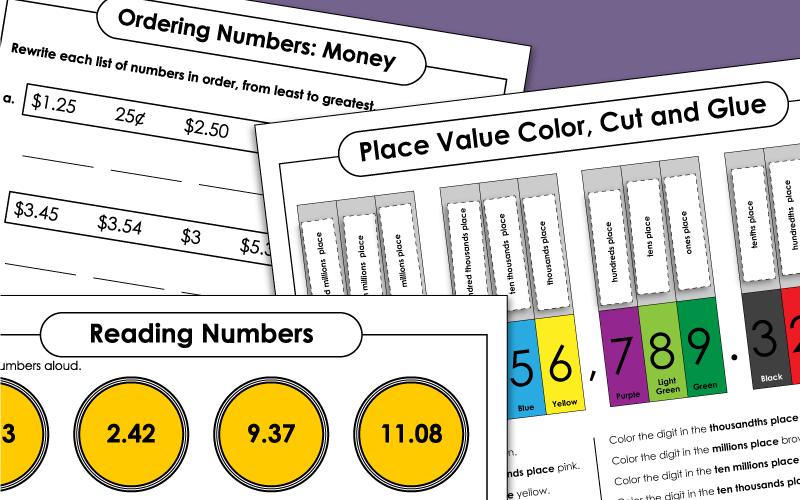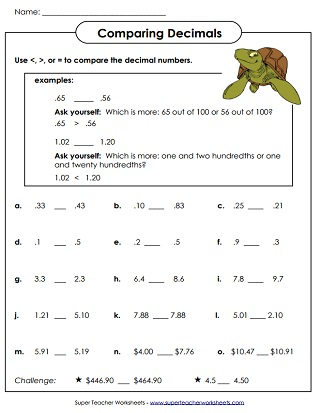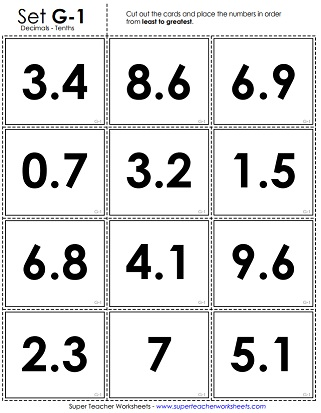# Place Value Decimals (Tenths)

The place value PDFs on this page can be used for teaching students to write numbers in word name format, standard notation, and expanded form. Also review ordering numbers, comparing numbers, and more.## Decimal Place Value

In the first part of this worksheet, students will write the value of the underlined digit. In the second half, they will answer several questions regarding the values of digits in a given number. Numbers go up to the tenths place.
Color each place correctly, according to the instructions. Then cut out the strips and glue each one in the correct position above the number.
Students are given digits.  Students arrange the digits to make the numbers given by the teacher.
Print out this anchor chart to show an example of standard form, written form, expanded form, and expanded notation of a number with digits to the tenths place.

Test your students on reading numbers with digits in the tenths place, as well as a few whole numbers to ensure they're reading the numbers closely. The file includes score sheets you can send home to parents as well.
Students must correctly read aloud the decimal amounts. Includes numbers with both tenths and hundredths.
Test your students' ability to read decimal numbers with this handy number wheel. The wheel includes 10 numbers with digits in the tenths place, as well as 2 whole 2-digit numbers to make sure they are reading each number carefully.

## Comparing Decimals

Practice comparing numbers with this worksheet, which has whole numbers, as well as numbers with values in the tenths place.

## Ordering Decimals

With this worksheet, students will be given groups of numbers and asked to rewrite them in order from least to greatest. Some numbers are whole, while others have digits in the tenths place.
This worksheet has whole numbers, decimals to the nearest tenth, and decimals to the nearest hundredth. Students determine their values and list them from least to greatest.
These cards have decimal numbers (tenths) on them.  Cut the cards and arrange them in order from least to greatest.
Decimal Place Value: Hundredths

Learn about basic decimals place value to the nearest tenths and hundredth.

## Worksheet ImagesMy Account
Site Information